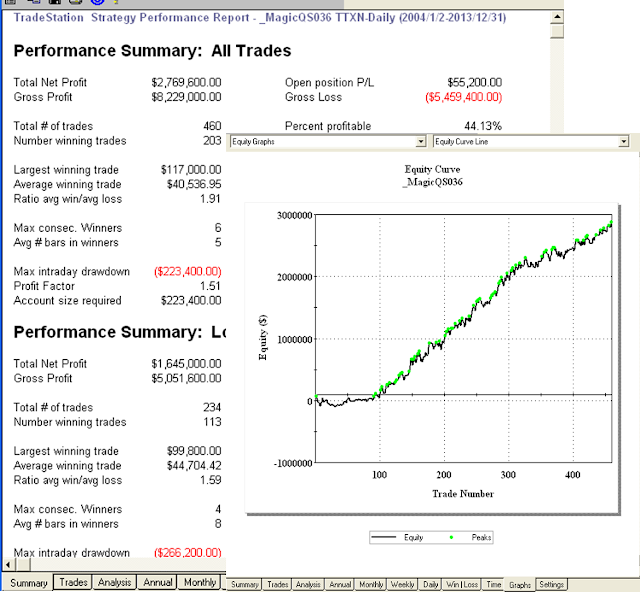## 2014年1月6日 星期一

### 選擇權籌碼在台指期交易策略的應用 (程式碼)

inputs: FastLen(5),SlowLen(21),AvgLen(9),SHB(1),SLB(1) ;
Vars: OI(0),OI1(0),OI2(0),PCEnergy(0),PCRatio(0),PutCng(0),CallCng(0),PCGap(0) ;

MP = MarketPosition ;
if DAYofMonth(Date) > 14 and DAYofMonth(Date) < 22 and DAYofWeek(Date)= 3 then isBalanceDay = True else isBalanceDay =False ;

{ Data3 OI1 賣權 OI2 買權 }
if Date <> Date then Begin
OI1 = Close of Data3 ;
OI2 = Volume of Data3 ;
PCEnergy = (OI1- OI2)/1000 ;
End;

if EntryType = 1 then Begin
if PCEnergy > {Cross over} HB then buy next bar at Market ;
if PCEnergy < {Cross under} LB then sell next bar at Market ;
end;

if EntryType = 2 then Begin
if PCEnergy > {Cross over} HB then Buy next bar at Highest(High,3) stop ;
if PCEnergy < {Cross under} LB then Sell next bar at Lowest(Low,3) stop ;
end;

SetStopLoss(PL * BigPointValue) ;

if ExitType = 1 then setProfitTarget(PF * BigPointValue) ;

if ExitType = 2 then Begin
if MP > 0 and BarsSinceEntry = NBarL then sell {ExitLong} next bar at Market ;
if MP < 0 and BarsSinceEntry = NBarS then Buy {ExitShort} next bar at Market ;
end;

if ExitType = 3 then Begin
SetProfitTarget(PF * BigPointValue) ;
if MP > 0 and BarsSinceEntry = NBarL then ExitLong next bar at Market ;
if MP < 0 and BarsSinceEntry = NBarS then ExitShort next bar at Market ;
end;

if IsBalanceDay then setExitonClose ;MagicQS036